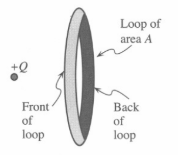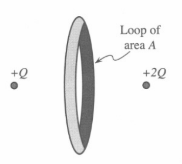# Problem: The loop shown at right has an area A.a. A positive charge with twice the value of the initial charge is placed to the right of the loop as shown. Both charges are the same distance from the loop and are placed along the axis of the loop. Is the net electric flux through the loop due to the charges positive, negative, or zero? Explain your reasoning.b. Suppose that the new charge located to the right of the loop had been negative instead of positive. How would your answer to part a change, if at all? Explain.

###### FREE Expert Solution

a.

The electric field is expressed as  E = kQ/r2.

Electric flux, ΦE = EA cos θ

Electric field strength depends on the magnitude of the charge.###### Problem Details

The loop shown at right has an area A.a. A positive charge with twice the value of the initial charge is placed to the right of the loop as shown. Both charges are the same distance from the loop and are placed along the axis of the loop.

Is the net electric flux through the loop due to the charges positive, negative, or zero? Explain your reasoning.

b. Suppose that the new charge located to the right of the loop had been negative instead of positive. How would your answer to part a change, if at all? Explain.